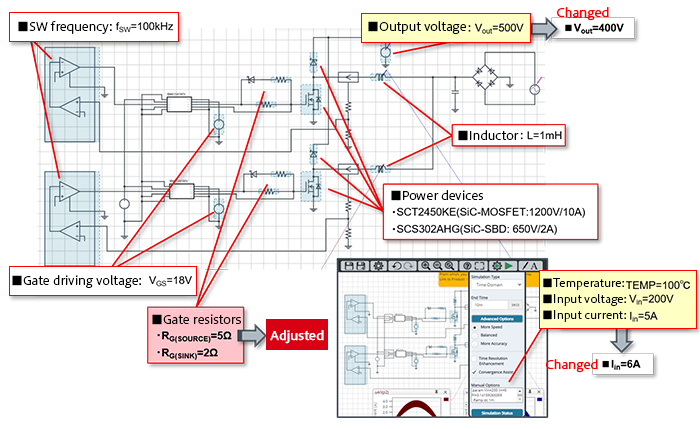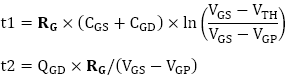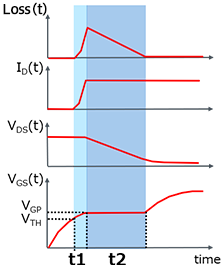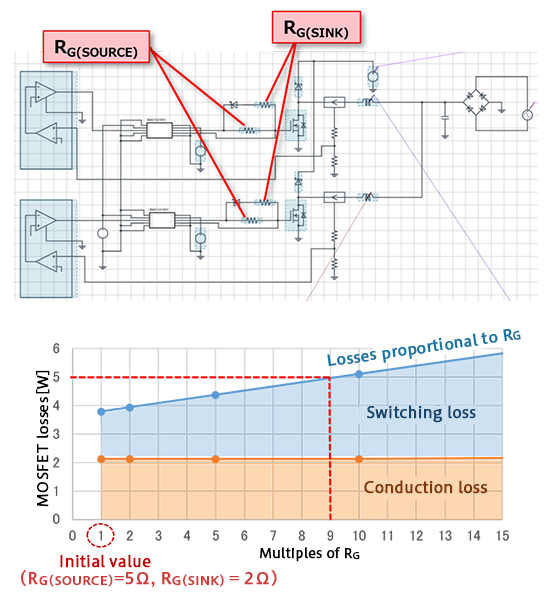# PFC Circuits: Changing the Gate Resistance

2021.08.18

・When the gate resistance of a switching element is increased, noise is suppressed, but there is a tradeoff with reduced efficiency. Hence it is extremely important that the gate resistance be set so as to achieve a balance.

・Simulations can be used to determine the maximum value of the gate resistance RG when holding switching element losses to below a specific value.

## PFC Circuits: Changing the Gate Resistance

In actual circuit design, noise reduction is a major issue, and in general noise is suppressed when the gate resistance of a switching element is increased. However, because there is a tradeoff due to the fact that efficiency is reduced (losses increase), it is extremely important that the gate resistance be set so as to balance the two. Here, the maximum gate resistance RG when switching element losses are held below a prescribed value is studied. To study noise, evaluations using actual equipment are necessary, and so here noise issues are not addressed.

### Example Circuit

As an example circuit, the simulation circuit “A-5. PFC CCM 2-PhaseVin=200V Iin=5A” in the Power Device Solution Circuit/AC-DC PFC list is used (see Fig.13). Details of the circuit diagram can also be seen here.

In this example, while holding losses in the SCT2450KE SiC MOSFET that is the low-side switching element in Fig. 13 to 5 W or less, we use simulations to study how high the gate resistance RG can be set in order to deal with noise.Fig. 13: PFC simulation circuit “A-5. PFC CCM 2-Phase Vin=200V Iin=5A”

### The Relationship Between Gate Resistance and Losses

Fig. 14 shows the relationship between losses, the drain current ID, the drain-source voltage VDS, and the gate voltage VGS when the SiC MOSFET is turned on. The periods t1 and t2 during which the switching loss occurs can be represented as follows.From these equations, we see that the times t1 and t2 at which switching losses occur are proportional to RG. Moreover, at these times ID and VDS change approximately linearly, and so losses can also be considered to be proportional to RG.Fig. 14: Relation between losses, ID, VDS, and VGS when turned on

### Adjustment of the Gate Resistance

Fig. 15 shows the results of simulation of SiC MOSFET losses when RG is changed. To avoid complexity, the resistance values for the source and the sink are changed in equal multiples.Fig. 15: SiC MOSFET loss simulation results when RG is changed

These simulation results show that, while the conduction loss is constant and unaffected by RG, the switching loss, as explained in “The Relationship Between Gate Resistance and Losses” above, is proportional to the value of RG. In order to hold losses to under 5 W, we see that RG must be held to within 9 times the initial value; that is, resistance values should be set such that RG(SOURCE) is 45 Ω or less, and RG(SINK) is 18 Ω or less.